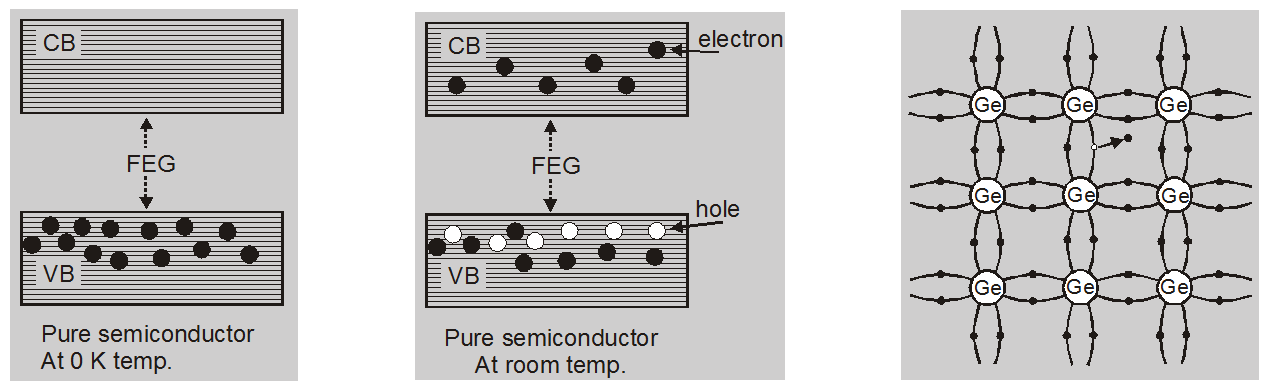Deepak Scored 45->99%ile with Bounce Back Crack Course. You can do it too!

# Effect of Temperature on semiconductor - Electronics - eSaral

Hey, do you want to learn about the Effect of Temperature on Semiconductor? If yes. Then keep reading.

## Effect of Temperature on Semiconductor

### At Absolute zero temperature

Covalent Bonds are very strong and there is no free electron in the conduction band. For semiconductors at 0 K, the conduction band is empty while the valence band is full.

### Above Absolute zero temperature

When temperature increases some of the covalent bonds are break due to thermal energy supplied.

At room temperature, due to thermal energy, some electrons jump to the conduction band.

In the conduction band electrons are free to move. If a potential difference is applied across semiconductor crystals, these free electrons constitute an electric current.

The variation in the resistivity of a pure semiconductor is mainly due to a change in carrier concentration.

The number of electrons raised from VB to the CB

$n_{e} \propto e^{-E_{o} / k T}$

$\mathbf{n}_{\mathrm{e}}=\mathrm{AT}^{3 / 2} \mathrm{e}^{-\Delta \mathrm{Eg} / 2 \mathrm{kT}}$

$\mathrm{T}=$ Absolute Temp.

$\mathrm{k}=$ Boltzmann Const. $=1.38 \times 10^{-23} \mathrm{~J} / \mathrm{K}$ As temperature (T) increases,

$\mathrm{n}_{\mathrm{e}}$ also increases

i.e. conductivity of the semiconductor increases.

As the temperature of the semiconductor increases, its resistivity decreases.

## Hole:

At higher temperatures, some of the electrons gain energy due to thermal agitation and move from the VB to the CB.

The electron leaving the VB to enter CB leave behind an equal number of vacant sites near the top of the VB. These vacant sites are called holes.These holes represent the vacancy of electrons and behave like a positive charge.

### Characteristics of hole:

• The hole carries a positive charge equal to an electronic charge.

• The energy of a hole is high as compared to that of the electron.

• The mobility of the hole is smaller than that of the electron.

• In the external electric field, holes move in a direction opposite of that of the electron.

• The effective mass of hole > Effective mass of the electron.

### Electron-hole Recombination:

The completion of a bond may not be necessarily due to an electron from a bond of a neighboring atom. The bond may be completed by a conduction band electron i.e., free electron, and known as electron-hole recombination.

The breaking of bonds or generation of electron-hole pairs and completion of bonds due to recombination is taking place all the time.

At equilibrium, the rate of generation becomes equal to the rate of recombination, giving a fixed number of free electrons and holes.

So, that's all from this article. I hope you get the idea about the Effect of Temperature on Semiconductor. If you found this article informative then please share it with your friends. If you have any confusion related to this topic, then you can ask in the comments section down below.

For a better understanding of this chapter, please check the detailed notes of Electronics. To watch Free Learning Videos on physics by Saransh Gupta sir Install the eSaral App.

Thank you for helping me am very glad for this website

#### Nikhil - April 1, 2022, 8:36 a.m.

Heating of device means its performance increase?

#### Zubair Ali - March 24, 2022, 11:21 p.m.

sir, can you briefly explain , when the temperature increase from 0 C to 90C of the semiconductor material (i.e Zinc Oxide) the conductivity increase, but up to some limit(100-110C) there conductivity gradually decrease.???

None
Free Study Material